Publicité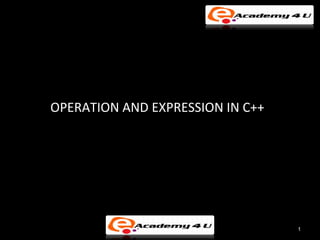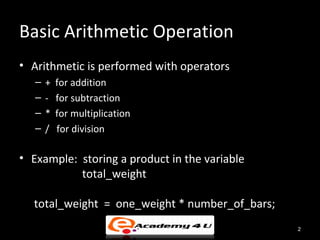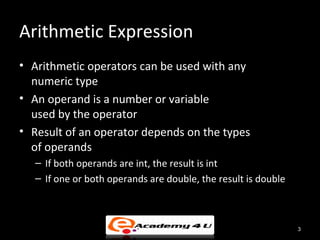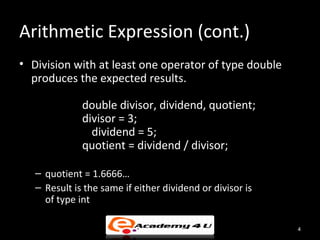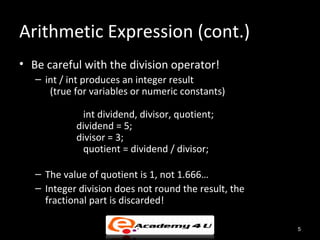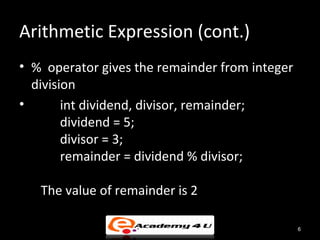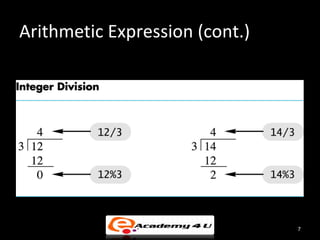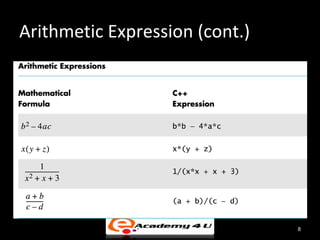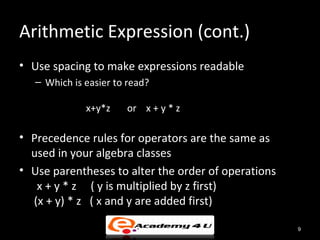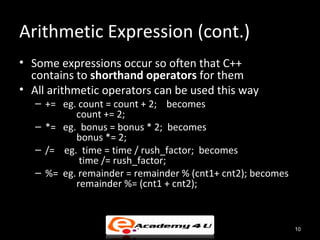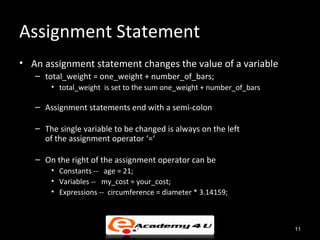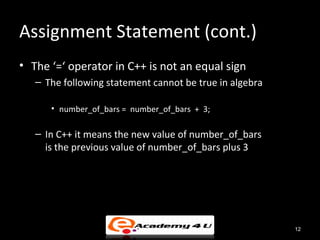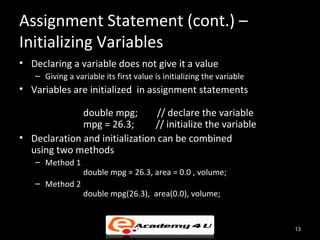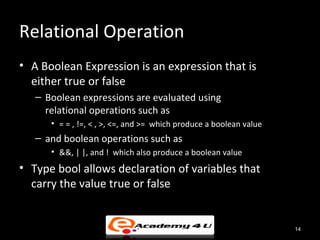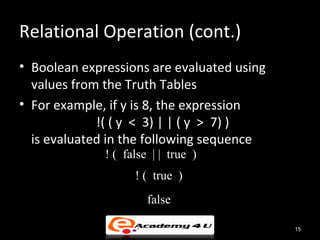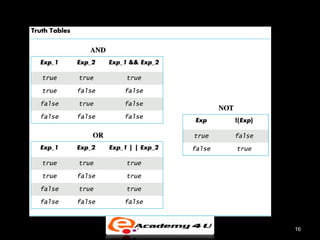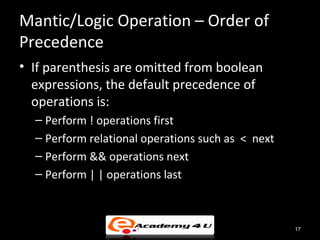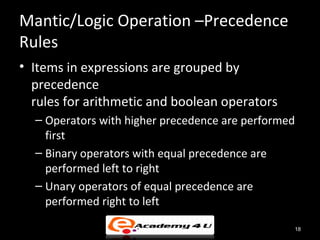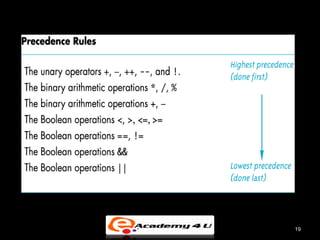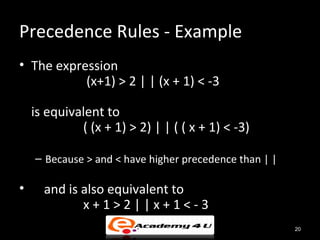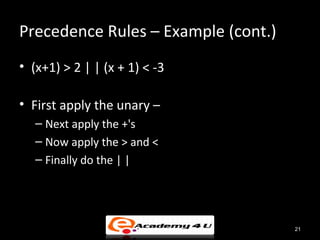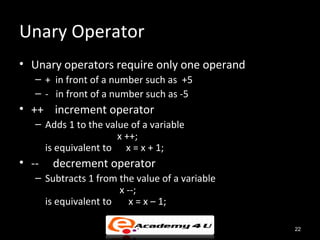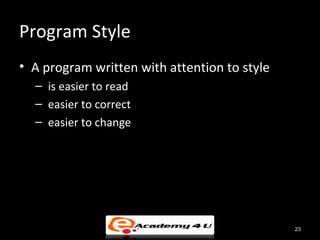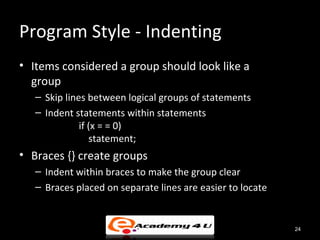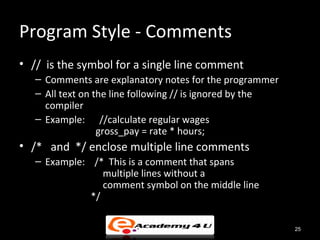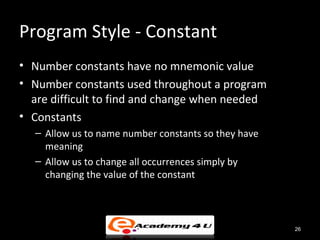1 sur 26
Publicité

### Operation and expression in c++

1. OPERATION AND EXPRESSION IN C++ 1
2. Basic Arithmetic Operation • Arithmetic is performed with operators – + for addition – - for subtraction – * for multiplication – / for division • Example: storing a product in the variable total_weight total_weight = one_weight * number_of_bars; 2
3. Arithmetic Expression • Arithmetic operators can be used with any numeric type • An operand is a number or variable used by the operator • Result of an operator depends on the types of operands – If both operands are int, the result is int – If one or both operands are double, the result is double 3
4. Arithmetic Expression (cont.) • Division with at least one operator of type double produces the expected results. double divisor, dividend, quotient; divisor = 3; dividend = 5; quotient = dividend / divisor; – quotient = 1.6666… – Result is the same if either dividend or divisor is of type int 4
5. Arithmetic Expression (cont.) • Be careful with the division operator! – int / int produces an integer result (true for variables or numeric constants) int dividend, divisor, quotient; dividend = 5; divisor = 3; quotient = dividend / divisor; – The value of quotient is 1, not 1.666… – Integer division does not round the result, the fractional part is discarded! 5
6. Arithmetic Expression (cont.) • % operator gives the remainder from integer division • int dividend, divisor, remainder; dividend = 5; divisor = 3; remainder = dividend % divisor; The value of remainder is 2 6
7. Arithmetic Expression (cont.) 7
8. Arithmetic Expression (cont.) 8
9. Arithmetic Expression (cont.) • Use spacing to make expressions readable – Which is easier to read? x+y*z or x + y * z • Precedence rules for operators are the same as used in your algebra classes • Use parentheses to alter the order of operations x + y * z ( y is multiplied by z first) (x + y) * z ( x and y are added first) 9
10. Arithmetic Expression (cont.) • Some expressions occur so often that C++ contains to shorthand operators for them • All arithmetic operators can be used this way – += eg. count = count + 2; becomes count += 2; – *= eg. bonus = bonus * 2; becomes bonus *= 2; – /= eg. time = time / rush_factor; becomes time /= rush_factor; – %= eg. remainder = remainder % (cnt1+ cnt2); becomes remainder %= (cnt1 + cnt2); 10
11. Assignment Statement • An assignment statement changes the value of a variable – total_weight = one_weight + number_of_bars; • total_weight is set to the sum one_weight + number_of_bars – Assignment statements end with a semi-colon – The single variable to be changed is always on the left of the assignment operator ‘=‘ – On the right of the assignment operator can be • Constants -- age = 21; • Variables -- my_cost = your_cost; • Expressions -- circumference = diameter * 3.14159; 11
12. Assignment Statement (cont.) • The ‘=‘ operator in C++ is not an equal sign – The following statement cannot be true in algebra • number_of_bars = number_of_bars + 3; – In C++ it means the new value of number_of_bars is the previous value of number_of_bars plus 3 12
13. Assignment Statement (cont.) – Initializing Variables • Declaring a variable does not give it a value – Giving a variable its first value is initializing the variable • Variables are initialized in assignment statements double mpg; // declare the variable mpg = 26.3; // initialize the variable • Declaration and initialization can be combined using two methods – Method 1 double mpg = 26.3, area = 0.0 , volume; – Method 2 double mpg(26.3), area(0.0), volume; 13
14. Relational Operation • A Boolean Expression is an expression that is either true or false – Boolean expressions are evaluated using relational operations such as • = = , !=, < , >, <=, and >= which produce a boolean value – and boolean operations such as • &&, | |, and ! which also produce a boolean value • Type bool allows declaration of variables that carry the value true or false 14
15. Relational Operation (cont.) • Boolean expressions are evaluated using values from the Truth Tables • For example, if y is 8, the expression !( ( y < 3) | | ( y > 7) ) is evaluated in the following sequence ! ( false | | true ) ! ( true ) false 15
16. 16
17. Mantic/Logic Operation – Order of Precedence • If parenthesis are omitted from boolean expressions, the default precedence of operations is: – Perform ! operations first – Perform relational operations such as < next – Perform && operations next – Perform | | operations last 17
18. Mantic/Logic Operation –Precedence Rules • Items in expressions are grouped by precedence rules for arithmetic and boolean operators – Operators with higher precedence are performed first – Binary operators with equal precedence are performed left to right – Unary operators of equal precedence are performed right to left 18
19. 19
20. Precedence Rules - Example • The expression (x+1) > 2 | | (x + 1) < -3 is equivalent to ( (x + 1) > 2) | | ( ( x + 1) < -3) – Because > and < have higher precedence than | | • and is also equivalent to x+1>2||x+1<-3 20
21. Precedence Rules – Example (cont.) • (x+1) > 2 | | (x + 1) < -3 • First apply the unary – – Next apply the +'s – Now apply the > and < – Finally do the | | 21
22. Unary Operator • Unary operators require only one operand – + in front of a number such as +5 – - in front of a number such as -5 • ++ increment operator – Adds 1 to the value of a variable x ++; is equivalent to x = x + 1; • -- decrement operator – Subtracts 1 from the value of a variable x --; is equivalent to x = x – 1; 22
23. Program Style • A program written with attention to style – is easier to read – easier to correct – easier to change 23
24. Program Style - Indenting • Items considered a group should look like a group – Skip lines between logical groups of statements – Indent statements within statements if (x = = 0) statement; • Braces {} create groups – Indent within braces to make the group clear – Braces placed on separate lines are easier to locate 24
25. Program Style - Comments • // is the symbol for a single line comment – Comments are explanatory notes for the programmer – All text on the line following // is ignored by the compiler – Example: //calculate regular wages gross_pay = rate * hours; • /* and */ enclose multiple line comments – Example: /* This is a comment that spans multiple lines without a comment symbol on the middle line */ 25
26. Program Style - Constant • Number constants have no mnemonic value • Number constants used throughout a program are difficult to find and change when needed • Constants – Allow us to name number constants so they have meaning – Allow us to change all occurrences simply by changing the value of the constant 26
Publicité